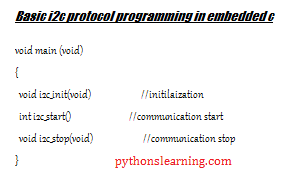# Basic i2c protocol programming in embedded c

In the previous article we saw basic communication protocol for embedded c so this time to implement real time projects on i2c protocol let’s see:

Table of Contents

### Follow below simple steps for i2c protocol programming

• Master start the communication by creating a Start condition
• Master sends address or response to the Slave,
• After receiving data or address, Slave generate an ACK /NACK,
• Master Check if slave generate ACK, then again Master sends data to the Slave,
• Again master check if slave generate NACK then Master closes the communication by creating a Stop condition.

### i2c protocol programming in embedded cBasic i2c protocol programming in embedded c

basically this code is dummy code or like syntax . we dived this code in two section

1. start condition
2. stop condition

before going toward we will see basic syntax of i2c protocol

``````void main (void)
{
void i2c_init(void)                         //initilaization
int i2c_start()                             //communication start
void i2c_stop(void)                         //communication stop
}``````

#### Start condition for i2c protocol

``````sbit SDA=P1^1; // initialize the SDA and SCL pins //
sbit SCL=P1^2;
void delay(unsigned int);
void main ()
{
SDA=1; //processing the data//
SCL=1; //clock is 1//
delay();
SDA=0; //sent the data//
delay();
SCL=0; //clock signal is 0//
}
Void delay(int p)
{
unsignedinta,b;
For(a=0;a<255;a++); //use delay function//
For(b=0;b<p;b++);
}``````

#### Stop condition for i2c protocol

``````void main ()
{
SDA=0; // Stop processing //
SCL=1; //clock is 1//
delay();
SDA=1; //Stopped//
delay();
SCL=0; //clock signal is 0//
}
Void delay(int p)
{
unsignedinta,b;
For(a=0;a<255;a++); //use delay function//
For(b=0;b<p;b++);
}``````

Sample code for i2c protocol

``````#include<lpc21xx.h>
#define AA 2
#define SI 3
#define STO 4
#define STA 5
#define I2EN 6

void wait(unsigned int delay)
{
while(delay--);
}

void i2c_init(void)       //I2C initilaization
{
I2SCLH=100;              //Bit frequency
I2SCLL=100;
I2CONSET=1<<I2EN;
}

int i2c_start()          //I2C communication
{
I2CONCLR=1<<3;
I2CONSET=1<<STA;
while(!(I2STAT==0x08));
return 0;
}

int i2c_write(unsigned char buff)      //Data writing through I2C
{
I2CONSET|=1<<SI;
I2DAT=buff;
wait(5000);
I2CONCLR=1<<SI;
wait(5000);
return 0;
}

void i2c_stop(void)       //end the I2C communication
{
I2CONSET=(1<<STO);
I2CONCLR=(1<<I2EN);
}

int main(void)
{
VPBDIV=0x02;             //select FCLK frequency
PINSEL0=1<<4|1<<6;       //select I2C communication pins
i2c_init();
i2c_start();
i2c_write(0x00);
i2c_write('A');
i2c_write('B');
i2c_write('C');
i2c_stop();
while(1);
}``````

Summary :

In this article we saw basic i2c protocol programming in embedded c so about this article you have any query then free to ask me

Also read :

Basic communication protocol in embedded c

### educational supportI am sach educational support Author on pythonslearning.com who love to share informative content on educational resources.